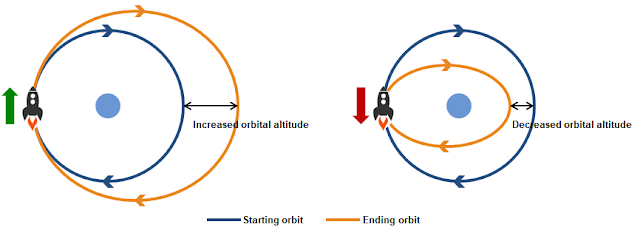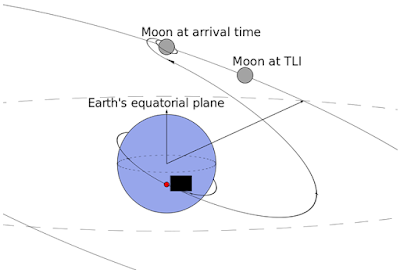### Section 4.4 - Types of Orbits and Orbital Maneuvers

#### Orbital Classifications

Now that we know how to define a given orbit, we can now classify different orbits based on some common characteristics, such as altitude, eccentricity, inclination, synchronicity, and what's being orbited, just to name a few. There are many more than what I'll show here, I'll just cover some key ones

Altitude:
• Low Earth Orbit (LEO): 160 - 2,000 km. A lot of objects orbit at LEO, such as the International Space Station. No human being has ventured beyond LEO since 1972, the last Apollo Moon mission
• Medium Earth Orbit (MEO): 2,000 - 35,786 km (cutoff for geosynchronous orbit, defined below)
• High Earth Orbit (HEO): >35,786 km

Eccentricity: Is the orbit close to circular or highly elliptical?

Inclination: These were introduced in the last section, but I'll repeat them here anyways
• Prograde orbit: $0^\circ < i < 90^\circ$ (moving eastward at ascending node)
• Polar orbit: $i = 90^\circ$ (moving due north at ascending node)
• Retrograde orbit: $90^\circ < i < 180^\circ$ (moving eastward at ascending node)

Prograde orbits are the most fuel efficient because launching eastward leverages the motion of Earth's rotation (for this reason, launching satellites to retrograde orbits is very rare). Polar orbits serve a very useful purpose in that they can cover the entirety of Earth's surface by orbiting in the North-South direction while the Earth rotates underneath it. See this video for a visualization

We'll discuss orbital inclination even more when we calculate launch azimuth and velocity in a later section.

Synchronicity: sometimes it's very useful to match orbital period (how long it takes the satellite to complete one orbit) with the rotational period of the body being orbited. For example:

• Geosynchronus Orbit (GSO): a satellite with an orbital period equal to exactly one Earth day (can be done with a circular orbit at an altitude of 35,786 km).
• Geostationary Orbit (GEO): a special and extremely useful type of GSO with an inclination of 0$^\circ$. A satellite in GEO will perfectly match the Earth's rotation and hover over the same point on Earth. From the ground, the satellite will appear like a fixed point in the sky. See below video

• Molniya Orbit: named after the Russian word for lightning, Molniya orbits are extremely popular with the Russians because they're an effective way to cover high altitudes. They are high-inclination semi-syncronous orbits (orbital period of half a day) which hang high above the Earth at apogee but quickly sweep past the low altitudes at perigee

As you can see, orbits can be categorized across many different criteria (for example, a medium altitude, highly elliptical, geosynchronous, polar orbit) and can be customized to suit a given application. Systems like GPS require a precisely choreographed constellation of satellites to effectively cover the entire globe at all times.

#### Orbital Maneuvers

Sometimes its necessary to make a course correction while in orbit. An on-orbit burn (ie a delta-v, for change in velocity) can alter the shape of an orbit.

Posigrade burns (forward in the direction of orbit) will raise the existing flight path, while retrograde burns (opposite the direction of orbit) will lower it.Left: Posigrade burn results in higher orbital altitude | Right: Retrograde burn lowers orbital altitude

An interesting feature of orbital burns is that if no other burn occurs, the spacecraft will always pass again through the point of burn. These burns can be combined into orbital maneuvers that will change the size, shape, or orientation of the orbit

Hohmann transfer: an elliptical orbit used to transfer between two circular orbits in the same plane. The first posigrade burn stretches the circular orbit into an elliptical one. Then at the apoapsis of the elliptical orbit, another posigrade burn circularizes the orbit at a higher altitude. Two retrograde burns can be done in the same order if a smaller circular orbit is desiredA Hohmann transfer orbit being used to increase orbital altitude

Bi-elliptic transfer: an alternate way to transfer between two circular orbits in the same plane. It takes a combination of three posigrade/retrograde burns (posigrade, posigrade, retrograde to increase, retrograde, retrograde, posigrade to decrease altitude), but despite the extra burn, it can actually be more fuel efficient than the Hohmann transfer if the ratio between the new and old altitudes is sufficiently high (~12x). A mathematical explanation for why is included in a later section.A bi-elliptic transfer being used to achieve a higher altitude circular orbit. Posigrade burns at points 1 and 2, retrograde burn at point 3

Orbital inclination changes: The Hohmann and bi-elliptic transfers are useful for orbits in the same plane, but what if we want to increase orbital inclination? These require much more energy, but can be done with an upward or downward thrust perpendicular to the existing orbital plane at one of the nodes. This will rotate the orbital plane about the line of nodesTo get from the initial orbit to the final, lower-inclination orbit, a downward thrust can be done at one of the nodes

#### Deep Space Maneuvers

The flight path the Apollo astronauts took to the Moon was approximately a Hohmann transfer that intersected the Moon and got caught in its gravity. This trajectory is initiated by a burn known as translunar injection, which is what initiates the Hohmann transfer.

The TLI puts the spacecraft into a highly elliptical orbit that's calculated such that apogee occurs right as the Moon passes by. Notice how the Moon's orbital plane is tilted relative to Earth's equatorial planeTLI would occur at the red dot

Another common maneuver used to accelerate a spacecraft without the use of fuel is called the gravitational slingshot. It leverages the gravity and the relative motion of a planet around the sun to alter the spacecraft's velocity. This has been used very successfully by many unmanned interplanetary probes

At first it's a bit confusing - as a spacecraft approaches the planet, its speed relative to the planet increases as it falls into its gravity well, but once it passes the planet and starts leaving, its speed decreases again as it works to escape the planet's gravity. If the net effect of this motion is 0, how does speed increase?A little bit of vector addition illustrates my point

The answer is that because of a planet's relative motion to the Sun, although the spacecraft's speed relative to the planet is the same before and after, its speed relative to the Sun increases